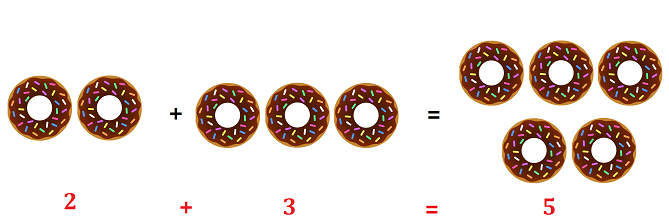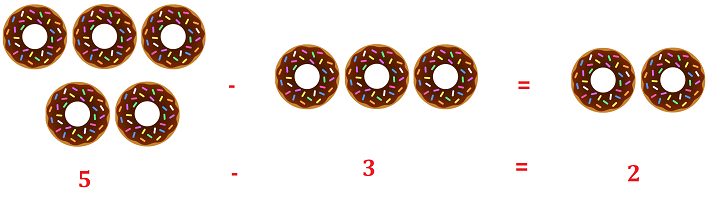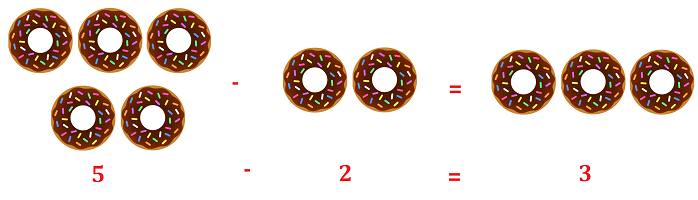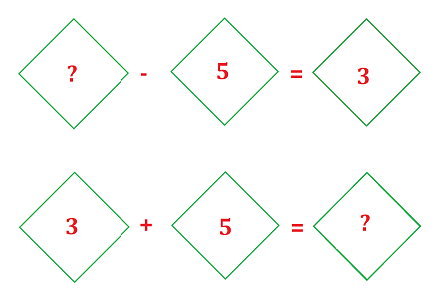# Math > Year 2

## Using inverse to solve missing number problems

What is Inverse?
Inverse means Opposite.
For example, High/Low, Hot/Cold, Big/Small etc.

What are Operations?
Operations in maths are addition, subtraction, multiplication and division.

What are Inverse Operations?
Inverse operations are operations that are opposite or “undo” each other.

Addition is the inverse of Subtraction and vice versa.

For example:The inverse of this addition equation is:ORMissing numbers and Inverse operations

We can use inverse operations to work missing numbers.

For example:So, the missing number is 8.

//